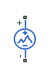# Piecewise Linear Voltage Source

Time-dependent voltage source using lookup table

• Library:
• Simscape / Electrical / Sources

Simscape / Electrical / Additional Components / SPICE Sources

•## Description

The Piecewise Linear Voltage Source block represents a voltage source that you specify in lookup table form using a vector of time values and a vector of the corresponding voltage values. You must specify at least two time-current value pairs. The block generates a time-dependent voltage based on these time-voltage values using the selected interpolation and extrapolation methods. You have a choice of two interpolation methods and extrapolation methods. The output voltage is independent of the current through the source.

## Ports

### Conserving

expand all

Electrical conserving port associated with the piecewise linear voltage source positive voltage

Electrical conserving port associated with the piecewise linear voltage source negative voltage

## Parameters

expand all

Vector of time values as a tabulated 1-by-n array. The time values vector must be strictly monotonically increasing.

Vector of voltage values as a tabulated 1-by-n array. The voltage values vector must be the same size as the time values vector.

Select the method that the block uses to determine the output current values at intermediate time points that are not specified in the preceding vectors:

• `Linear` — Prioritize performance by using a linear function.

• `Smooth` — Prioritize accuracy by producing a continuous curve with continuous first-order derivatives.

Select the method that the block uses to determine the output current values at time points that are outside the time range specified in the preceding vectors:

• `Nearest` — Select this option to use the nearest input value for extrapolation.

• `Linear` — Select this option to use a linear function.

 D. Kahaner, C. Moler, and S. Nash. Numerical Methods and Software . Prentice Hall, 1988.

 W.H. Press, B.P. Flannery, S.A. Teulkolsky, and W.T. Wetterling. Numerical Recipes in C: The Art of Scientific Computing . Cambridge University Press, 1992.